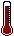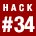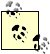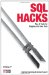# Hack 34. Calculate the MedianThe AVG function returns the arithmetic mean of a set of values. Sometimes the median value is a more appropriate "average."

When you want the average of a set of numbers with a long tail, the median can be more representative. Consider the time it takes to complete a task, as shown in Table 5-14.

Table 5-14. Time required to complete a task

subject minutes
Lisa 1
Marge 2
Bart 3
Homer 4
Ralph 90

The mean of these values is 20; it has been driven upward by a single outlier, Ralph. In this case, Bart's value of 3 is the median and it is more meaningful.

To calculate the median you need to find the middle row. This row represents the individual who completes a task faster than half of the population and slower than the other half.Actually the middle individual can't be faster than exactly half the population. He is faster than (population-1)/2 for an odd-size population. For an even-size population there are two middle individuals, and the median can be defined as the mean of these two.

For each subject you can show the number of people who are faster by running a subquery on the SELECT line. This is an unusual pattern, but it is perfectly legal and it works on SQL Server, Oracle, and MySQL:

```mysql> SELECT subject,
-> minutes,
-> (SELECT COUNT(1) FROM task y
-> WHERE y.minutes
+---------+---------+---------+
| subject | minutes | quicker |
+---------+---------+---------+
| Lisa | 1 | 0 |
| Marge | 2 | 1 |
| Bart | 3 | 2 |
| Homer | 4 | 3 |
| Ralph | 90 | 4 |
+---------+---------+---------+```

Given that there are five people in the list, you need to find the one who is in the middle; that is the person who was faster than two people and slower than two people. If you make the preceding query a subquery of another, you receive this:

```mysql> SELECT minutes FROM
-> (
-> SELECT subject,
-> minutes,
-> (SELECT COUNT(1) FROM task y
-> WHERE y.minutes
-> ) t
-> WHERE t.quicker = FLOOR((SELECT COUNT(*) FROM task)/2);
+---------+
| minutes |
+---------+
| 3 |
+---------+```

Sadly, this works only if the values are distinct. There might not be a person who is faster than half the population; for example, if the times in the table were 1, 1, 1, 1, and 96, for four people no one would be faster and four people would still be faster than Ralph. No one would have exactly two people faster than they are. The other problem is that the query is inefficient, so it will complete in quadratic time.

5.11.1. Create a Temporary Table

You can solve a load of problems with a temporary table. This is one of the occasions when a little bit of code will give better performance.

You can create a table and mark it as temporary with the following command:

```CREATE TEMPORARY TABLE taskI
(posn INTEGER
,subject VARCHAR(10)
,minutes INTEGER
)```

With a temporary table you can be certain that the table contents are visible only to the current session, so there is no danger of other processes interfering with your calculations.MySQL and PostgreSQL use the syntax shown. In Oracle, you use the phrase CREATE GLOBAL TEMPORARY TABLE, and in SQL Server, you use a # to indicate that the table is temporary, as in CREATE TABLE #taskI.

You need a table with a posn column that starts at 1 and increments, as shown in Table 5-15.

Posn subject time
1 Lisa 1
2 Marge 2
3 Bart 3
4 Homer 4
5 Ralph 90

5.11.2. Fill the Temporary Table

You can fill the table used to hold the positions with sequential values, using the technique shown in "Generate Sequential or Missing Data" [Hack #82] or using the methods shown in "Generate Unique Sequential Numbers" [Hack #57]. It is the same across all platforms, but the method used to fill it is different in each case.

Alternatively, you can write a little code to fill in those sequences, as explained in the following sections.

5.11.2.1. MySQL

In MySQL, you can update a variable in a SELECT statement. The first SELECT sets the local variable; the second SELECT uses it and updates it:

```SELECT @rownum:=0;
SELECT @rownum:=@rownum+1 rownum, subject, minutes

5.11.2.2. SQL Server

The variables in SQL Server look similar, but you may not reference the variable and update it in the same SELECT:

```INSERT INTO taskI(subject,minutes)
SELECT subject, minutes
ORDER BY minutes;
DECLARE @rownum AS INTEGER
SELECT @rownum=0
SET @rownum=@rownum+1,
posn = @rownum;```

5.11.2.3. Oracle

In Oracle, you don't actually need to create the table explicitly. However, having an actual table with an index can be faster. The pseudovariable ROWNUM gives the position of each row:

```INSERT INTO taskI
SELECT ROWNUM, subject, minutes
FROM (SELECT subject, minutes

5.11.3. Find the Middle Row or Rows

When the number of rows is even, one convention defines the median as the mean of the two middle elements. You need a query that gives the middle element when there are an odd number of rows and the mean of the middle two otherwise. You can do this with a little fancy integer arithmetic:

```mysql> SELECT AVG(minutes) FROM taskI,
-> (SELECT COUNT(*) n FROM task) t
-> WHERE posn IN (FLOOR((n+1)/2), FLOOR(n/2)+1);
+--------------+
| AVG(minutes) |
+--------------+
| 3.0000 |
+--------------+```

Here n is the number of rows; when n is odd both FLOOR((n+1)/2) and FLOOR(n/2)+1 evaluate to (n + 1) / 2. When n is even FLOOR((n+1)/2) evaluates to n / 2 and FLOOR((n+1)/2) evaluates to n / 2 + 1. Table 5-16 shows some applications of this calculation.

Table 5-16. Choosing the middle element(s)

sample n Middle element(s) FLOOR((n+1)/2) FLOOR(n/2)+1
1 2 3 3 2 2 2
1 2 3 4 4 2 3 2 3
1 2 3 4 5 5 3 3 3
1 2 3 4 5 6 6 3 4 3 4

### Hack 35 Tally Results into a ChartSQL Hacks
ISBN: 0596527993
EAN: 2147483647
Year: 2004
Pages: 147